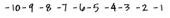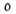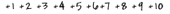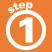Home    |    Teacher    |    Parents    |    Glossary    |    About UsAn integer is a whole number that can be either greater than 0, called positive, or less than 0, called negative. Zero is neither positive nor negative.

Two integers that are the same distance from zero in opposite directions are called opposites.

Every integer on the number line has an absolute value, which is its distance from zero.

Move your mouse cursor over the number line below to see its parts.Homework Help | Pre-Algebra | NumbersEmail this page to a friendSearch·  Place value·  Decimal numbers·  Estimating and     rounding·  Adding / subtracting     decimals·  Multiplying decimals·  Dividing decimals·  Percent·  Exponents·  Square roots·  Signed integers·  Adding and     subtracting integers·  Multiplying and     dividing integers·  Properties of integersFirst Glance In Depth Examples WorkoutSigned Integers